Area between curve and line

Chapter 8 Class 12 Application of Integrals
Concept wise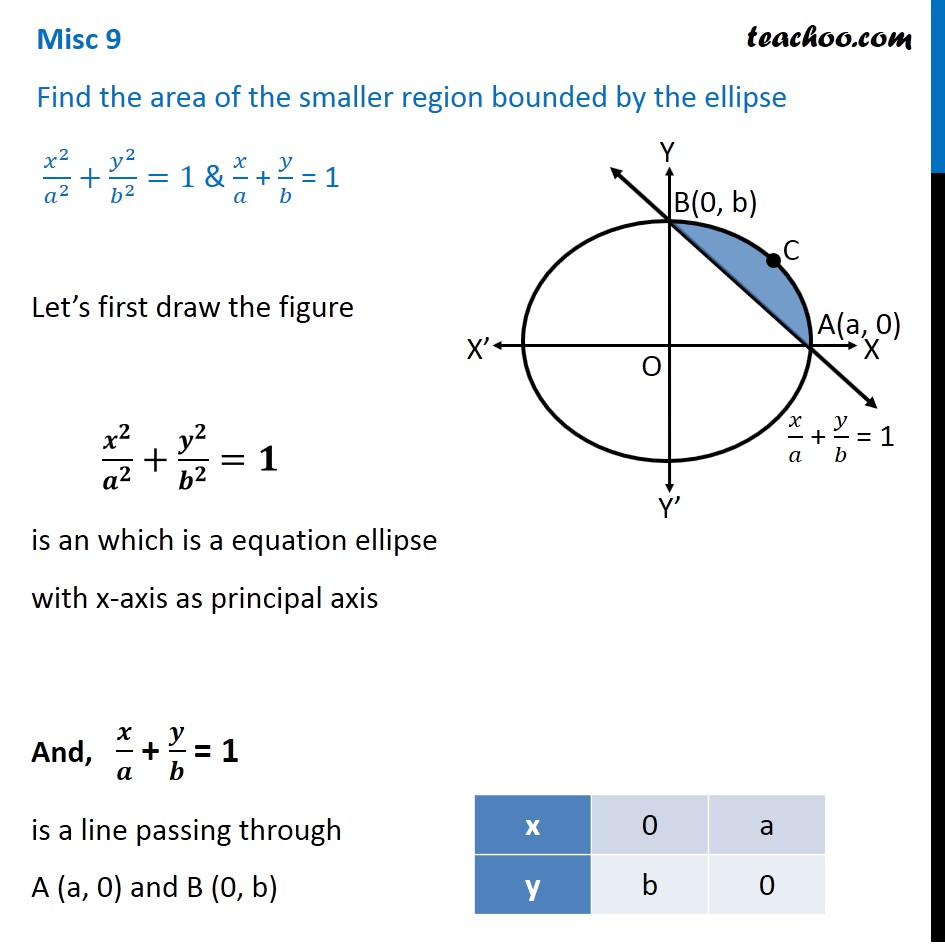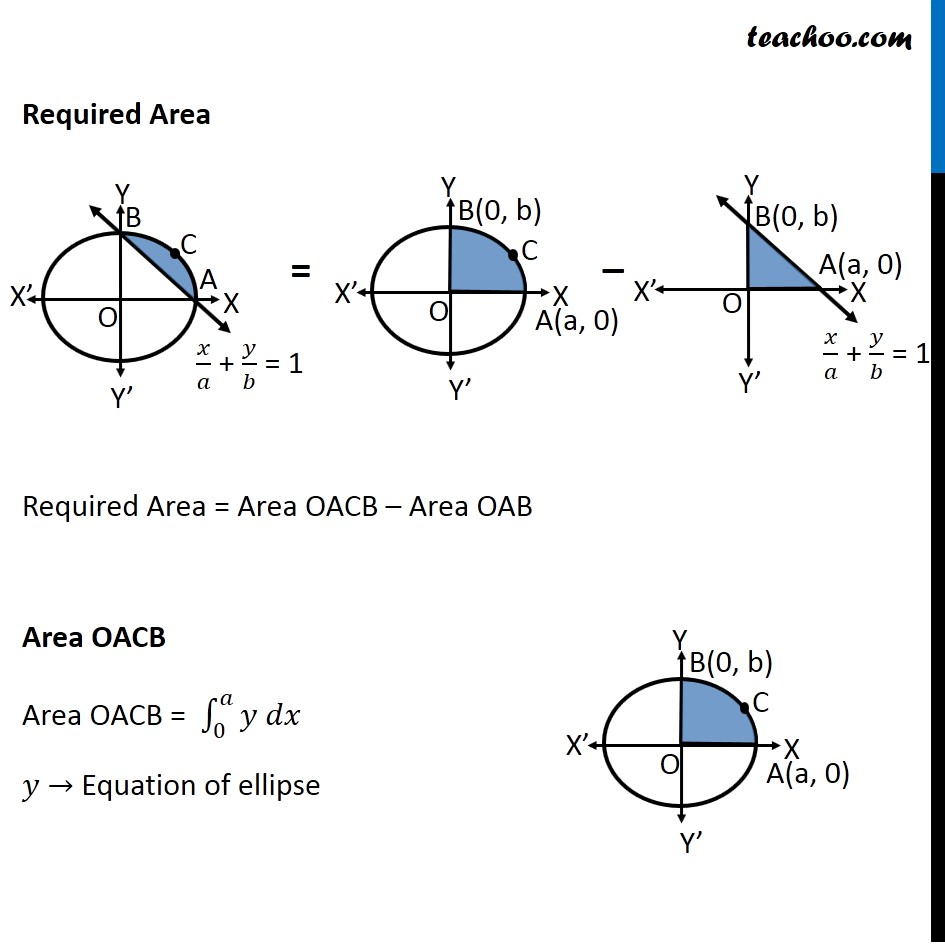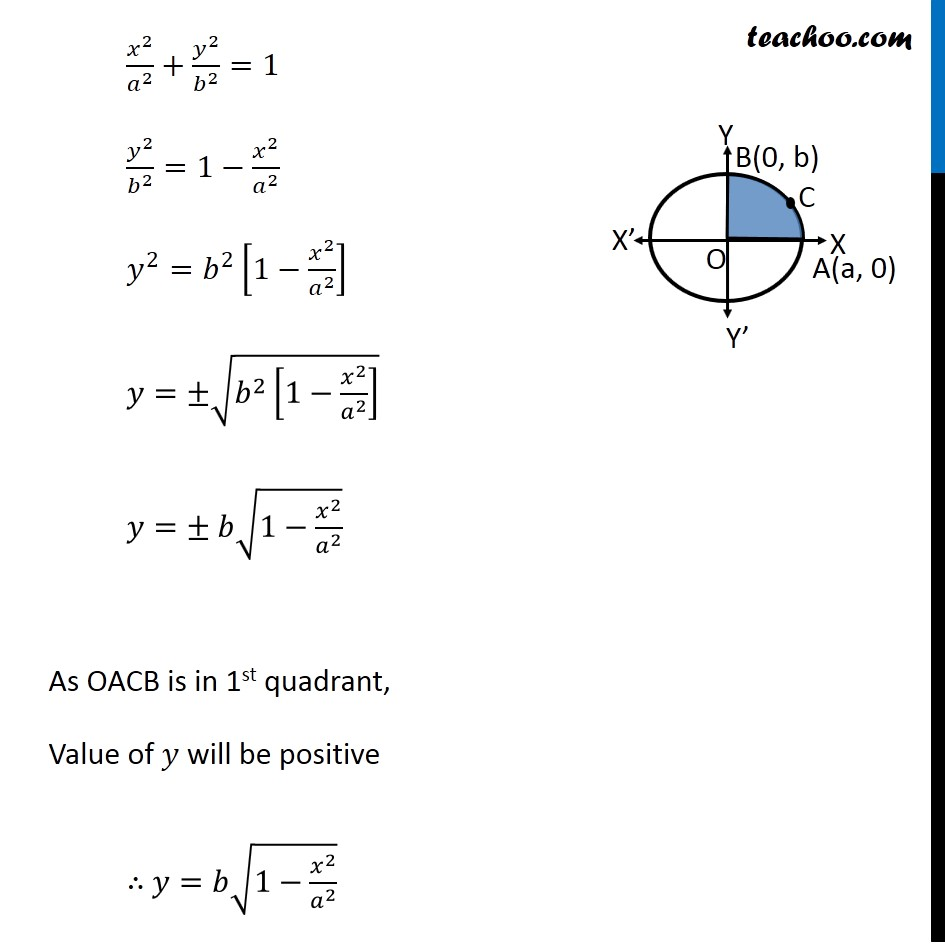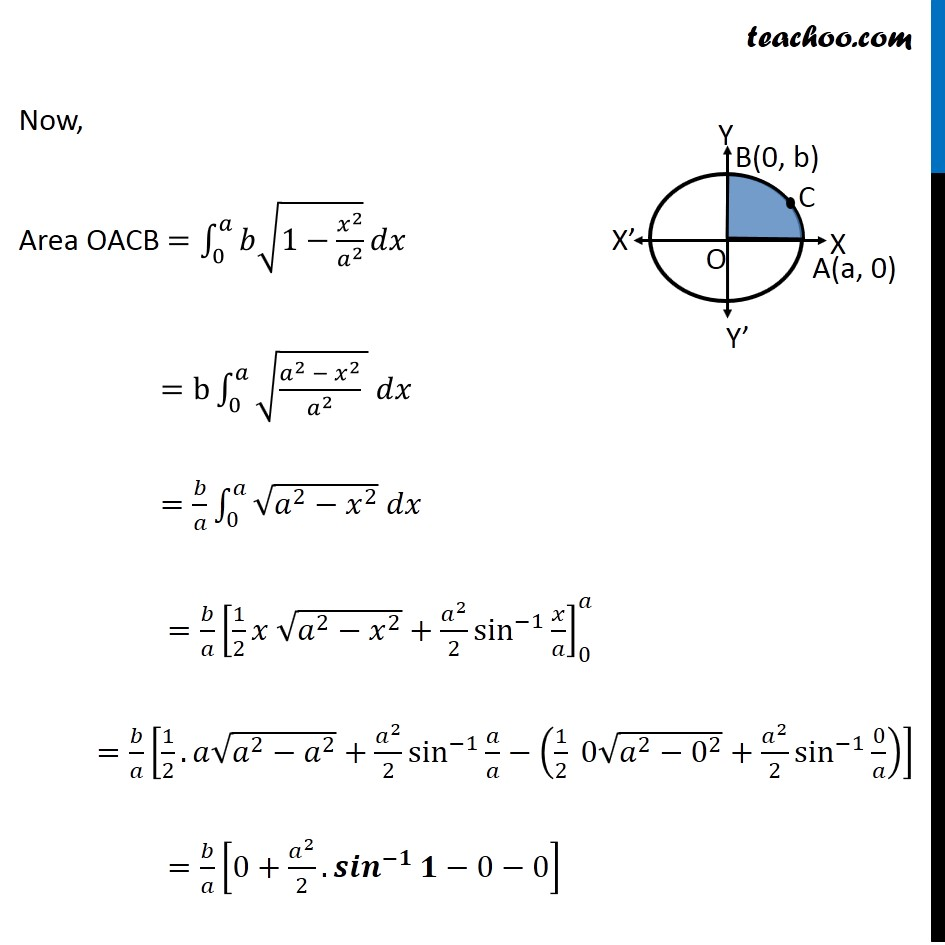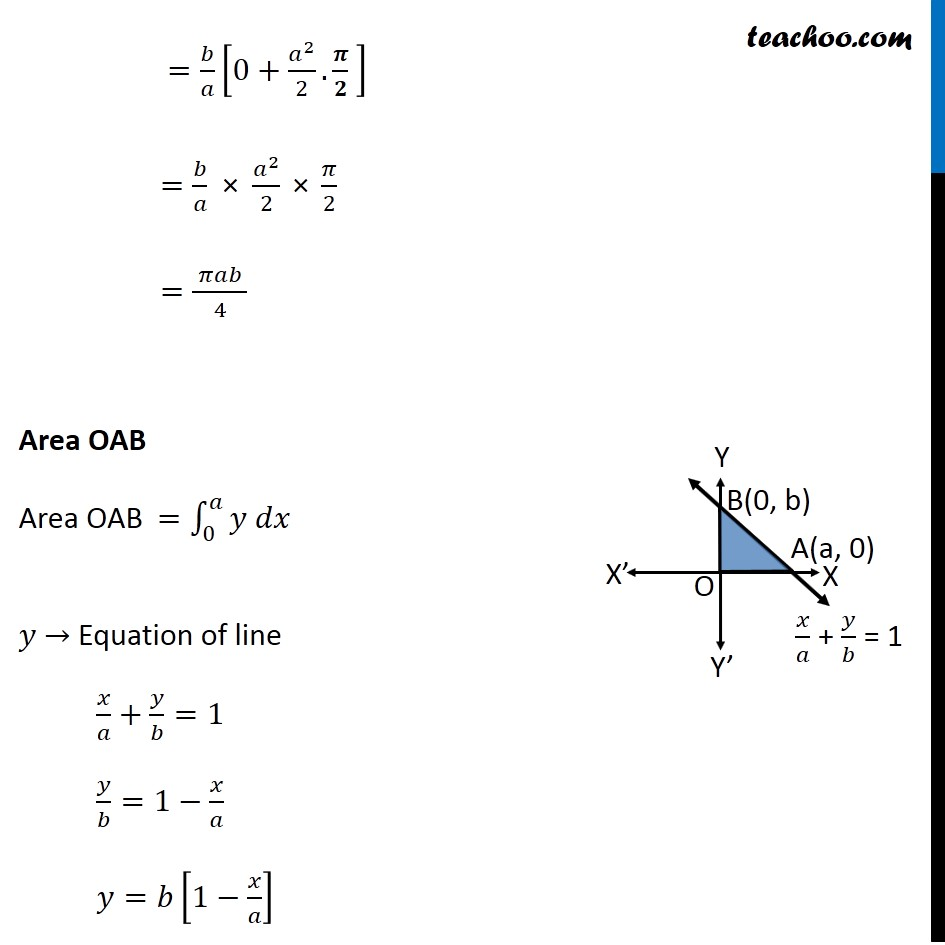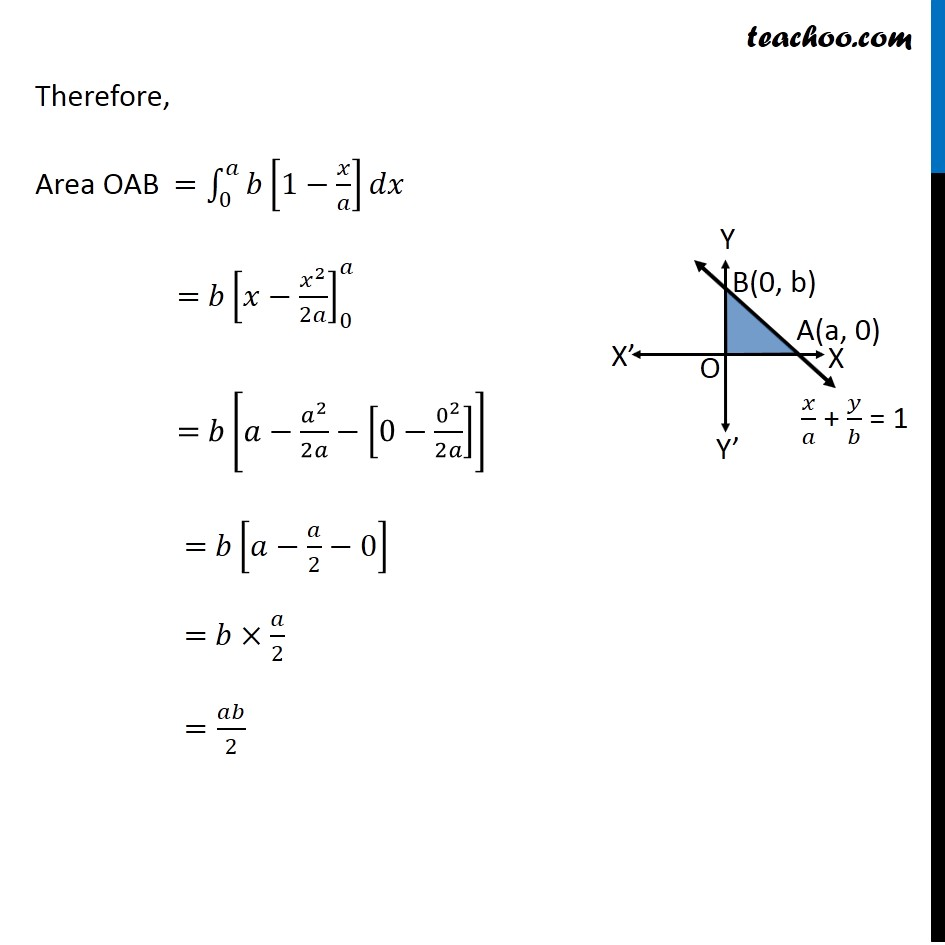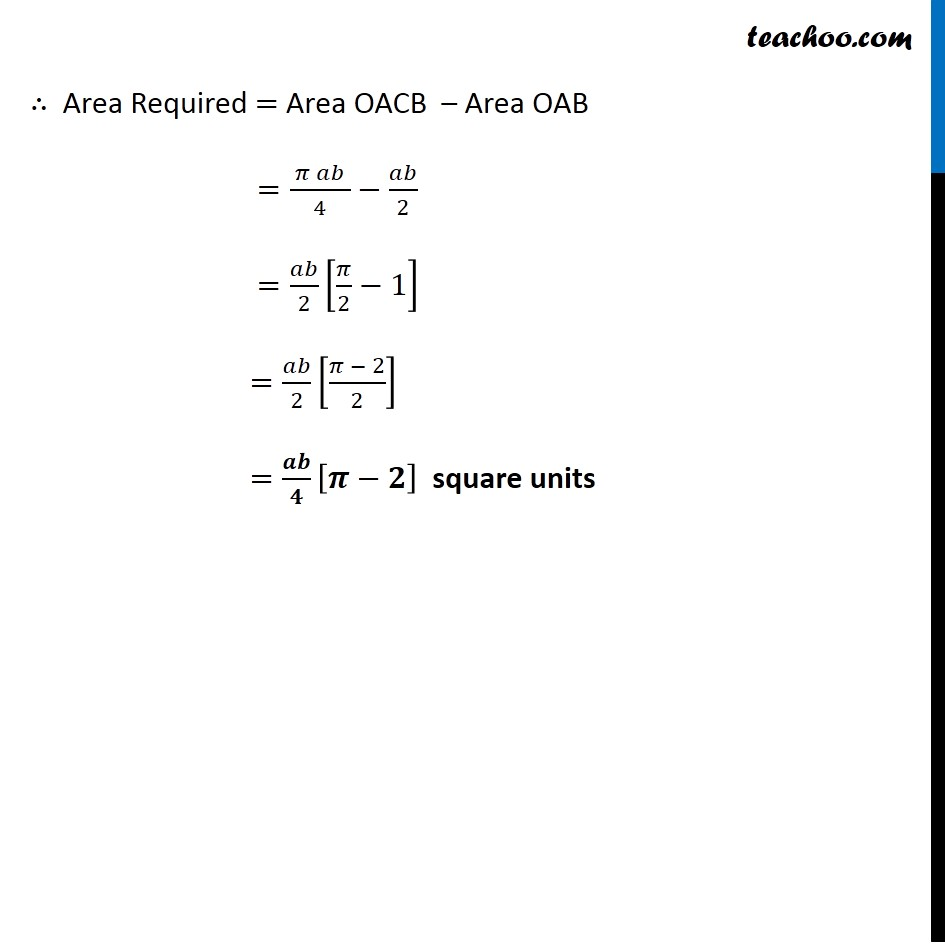Introducing your new favourite teacher - Teachoo Black, at only ₹83 per month

### Transcript

Misc 9 Find the area of the smaller region bounded by the ellipse 𝑥^2/𝑎^2 +𝑦^2/𝑏^2 =1 & 𝑥/𝑎 + 𝑦/𝑏 = 1 Let’s first draw the figure 𝒙^𝟐/𝒂^𝟐 +𝒚^𝟐/𝒃^𝟐 =𝟏 is an which is a equation ellipse with x-axis as principal axis And, 𝒙/𝒂 + 𝒚/𝒃 = 1 is a line passing through A (a, 0) and B (0, b) Required Area Required Area = Area OACB – Area OAB Area OACB Area OACB = ∫_0^𝑎▒〖𝑦 𝑑𝑥〗 𝑦 → Equation of ellipse 𝑥^2/𝑎^2 +𝑦^2/𝑏^2 =1 𝑦^2/𝑏^2 =1−𝑥^2/𝑎^2 𝑦^2=𝑏^2 [1−𝑥^2/𝑎^2 ] 𝑦=±√(𝑏^2 [1−𝑥^2/𝑎^2 ] ) 𝑦=± 𝑏√(1−𝑥^2/𝑎^2 ) As OACB is in 1st quadrant, Value of 𝑦 will be positive ∴ 𝑦=𝑏√(1−𝑥^2/𝑎^2 ) Now, Area OACB =∫_0^𝑎▒〖𝑏√(1−𝑥^2/𝑎^2 )〗 𝑑𝑥 =b∫_0^𝑎▒〖√((𝑎^2 − 𝑥^2)/𝑎^2 ) 𝑑𝑥" " 〗 =𝑏/𝑎 ∫_0^𝑎▒〖√(𝑎^2−𝑥^2 ) 𝑑𝑥" " 〗 =𝑏/𝑎 [1/2 𝑥 √(𝑎^2−𝑥^2 )+𝑎^2/2 sin^(−1)⁡〖𝑥/𝑎〗 ]_0^𝑎 =𝑏/𝑎 [1/2.𝑎√(𝑎^2−𝑎^2 )+𝑎^2/2 sin^(−1)⁡〖𝑎/𝑎〗−(1/2 0√(𝑎^2−0^2 )+𝑎^2/2 sin^(−1)⁡〖0/𝑎〗 )] =𝑏/𝑎 [0+𝑎^2/2.〖𝒔𝒊𝒏〗^(−𝟏)⁡𝟏−0−0] =𝑏/𝑎 [0+𝑎^2/2.𝝅/𝟐 ] =𝑏/𝑎 × 𝑎^2/2 " × " 𝜋/2 =( 𝜋𝑎𝑏 )/4 =𝑏/𝑎 [0+𝑎^2/2.𝝅/𝟐 ] =𝑏/𝑎 × 𝑎^2/2 " × " 𝜋/2 =( 𝜋𝑎𝑏 )/4 Area OAB Area OAB =∫_0^𝑎▒〖𝑦 𝑑𝑥〗 𝑦 → Equation of line 𝑥/𝑎+𝑦/𝑏=1 𝑦/𝑏=1−𝑥/𝑎 𝑦=𝑏[1−𝑥/𝑎] Therefore, Area OAB =∫_0^𝑎▒𝑏[1−𝑥/𝑎]𝑑𝑥 = 〖𝑏[𝑥−𝑥^2/2𝑎]〗_0^𝑎 = 𝑏[𝑎−𝑎^2/2𝑎−[0−0^2/2𝑎]] = 𝑏[𝑎−𝑎/2−0] = 𝑏×𝑎/2 =𝑎𝑏/2 ∴ Area Required = Area OACB – Area OAB =( 𝜋 𝑎𝑏 )/4−𝑎𝑏/2 =𝑎𝑏/2 [𝜋/2−1] =𝑎𝑏/2 [(𝜋 − 2)/2] =𝒂𝒃/𝟒 [𝝅−𝟐] square units